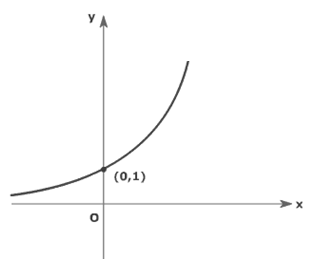2015 Countdown Problem #22: The Curve

Geometry Level 1The image shows a part of a graph. Below are four equations:

I: $y=\frac{2015}{e^x}$

II: $y=2015{e}^x$

III: $y={2015}^x$

IV:$y=e^{2015x}$

Which equation(s), when plotted, will produce the graph above?

This problem is part of the set 2015 Countdown Problems.

×